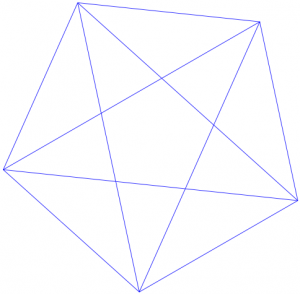$$\newcommand{\id}{\mathrm{id}}$$ $$\newcommand{\Span}{\mathrm{span}}$$ $$\newcommand{\kernel}{\mathrm{null}\,}$$ $$\newcommand{\range}{\mathrm{range}\,}$$ $$\newcommand{\RealPart}{\mathrm{Re}}$$ $$\newcommand{\ImaginaryPart}{\mathrm{Im}}$$ $$\newcommand{\Argument}{\mathrm{Arg}}$$ $$\newcommand{\norm}{\| #1 \|}$$ $$\newcommand{\inner}{\langle #1, #2 \rangle}$$ $$\newcommand{\Span}{\mathrm{span}}$$

# 1.5: Problem Bank

•• Contributed by Michelle Manes
• Professor (Mathematics) at University of Hawaii
$$\newcommand{\vecs}{\overset { \rightharpoonup} {\mathbf{#1}} }$$ $$\newcommand{\vecd}{\overset{-\!-\!\rightharpoonup}{\vphantom{a}\smash {#1}}}$$$$\newcommand{\id}{\mathrm{id}}$$ $$\newcommand{\Span}{\mathrm{span}}$$ $$\newcommand{\kernel}{\mathrm{null}\,}$$ $$\newcommand{\range}{\mathrm{range}\,}$$ $$\newcommand{\RealPart}{\mathrm{Re}}$$ $$\newcommand{\ImaginaryPart}{\mathrm{Im}}$$ $$\newcommand{\Argument}{\mathrm{Arg}}$$ $$\newcommand{\norm}{\| #1 \|}$$ $$\newcommand{\inner}{\langle #1, #2 \rangle}$$ $$\newcommand{\Span}{\mathrm{span}}$$ $$\newcommand{\id}{\mathrm{id}}$$ $$\newcommand{\Span}{\mathrm{span}}$$ $$\newcommand{\kernel}{\mathrm{null}\,}$$ $$\newcommand{\range}{\mathrm{range}\,}$$ $$\newcommand{\RealPart}{\mathrm{Re}}$$ $$\newcommand{\ImaginaryPart}{\mathrm{Im}}$$ $$\newcommand{\Argument}{\mathrm{Arg}}$$ $$\newcommand{\norm}{\| #1 \|}$$ $$\newcommand{\inner}{\langle #1, #2 \rangle}$$ $$\newcommand{\Span}{\mathrm{span}}$$

You have several problem solving strategies to work with. Here are the ones we have described so far (and you probably came up with even more of your own strategies as you worked on problems).

Try your hand at some of these problems, keeping these strategies in mind. If you are stuck on a problem, come back to this list and ask yourself which of the strategies might help you make some progress.

Problem 6

You have eight coins and a balance scale. The coins look alike, but one of them is a counterfeit. The counterfeit coin is lighter than the others. You may only use the balance scale two times. How can you find the counterfeit coin?Problem 7

You have five coins, no two of which weigh the same. In seven weighings on a balance scale, can you put the coins in order from lightest to heaviest? That is, can you determine which coin is the lightest, next lightest, . . . , heaviest.

Problem 8

You have ten bags of coins. Nine of the bags contain good coins weighing one ounce each. One bag contains counterfeit coins weighing 1.1 ounces each. You have a regular (digital) scale, not a balance scale. The scale is correct to one-tenth of an ounce. In one weighing, can you determine which bag contains the bad coins?

Problem 9

Suppose you have a balance scale. You have three different weights, and you are able to weigh every whole number from 1 gram to 13 grams using just those three weights. What are the three weights?

Problem 10

There are a bunch of coins on a table in front of you. Your friend tells you how many of the coins are heads-up. You are blindfolded and cannot see a thing, but you can move the coins around, and you can flip them over. However, you cannot tell just by feeling them if the coins are showing heads or tails. Your job: separate the coins into two piles so that the same number of heads are showing in each pile.

Problem 11

The digital root of a number is the number obtained by repeatedly adding the digits of the number. If the answer is not a one-digit number, add those digits. Continue until a one-digit sum is reached. This one digit is the digital root of the number.

For example, the digital root of 98 is 8, since 9 + 8 = 17 and 1 + 7 = 8.

Record the digital roots of the first 30 integers and find as many patterns as you can. Can you explain any of the patterns?

Problem 12

If this lattice were continued, what number would be directly to the right of 98? How can you be sure you’re right?

 3 6 9 12 … 1 2 4 5 7 8 10 11 13 …

Problem 13

Arrange the digits 0 through 9 so that the first digit is divisible by 1, the first two digits are divisible by 2, the first three digits are divisible by 3, and continuing until you have the first 9 digits divisible by 9 and the whole 10-digit number divisible by 10.

Problem 14

There are 25 students and one teacher in class. After an exam, everyone high-fives everyone else to celebrate how well they did. How many high- fives were there?

Problem 15

In cleaning out your old desk, you find a whole bunch of 3¢ and 7¢ stamps. Can you make exactly 11¢ of postage? Can you make exactly 19¢ of postage? What is the largest amount of postage you cannot make?

Problem 16

Find the largest eight-digit number made up of the digits 1, 1, 2, 2, 3, 3, 4, and 4 such that the 1’s are separated by one digit, the 2’s are separated by two digits, the 3’s by three digits, and the 4’s by four digits.

Problem 17

Kami has ten pockets and 44 dollar bills. She wants to have a different amount of money in each pocket. Can she do it?

Problem 18

How many triangles of all possible sizes and shapes are in this picture?Problem 19

Arrange the digits 1–6 into a “difference triangle” where each number in the row below is the difference of the two numbers above it.

Example: Below is a difference triangle, but it does not work because it uses 1 twice and does not have a 6:

 4 5 3 1 2 1

Problem 20

Certain pipes are sold in lengths of 6 inches, 8 inches, and 10 inches. How many different lengths can you form by attaching three sections of pipe together?

Problem 21

Place the digits 1, 2, 3, 4, 5, 6 in the circles so that the sum on each side of the triangle is 12. Each circle gets one digit, and each digit is used exactly once.Problem 22

Find a way to cut a circular pizza into 11 pieces using just four straight cuts